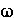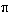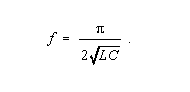Back

## resonance

In an electrical circuit, the condition that exists when the inductive reactance and the capacitive reactance are of equal magnitude, causing electrical energy to oscillate between the magnetic field of the inductor and the electric field of the capacitor. Note 1: Resonance occurs because the collapsing magnetic field of the inductor generates an electric current in its windings that charges the capacitor and the discharging capacitor provides an electric current that builds the magnetic field in the inductor, and the process is repeated. Note 2: At resonance, the series impedance of the two elements is at a minimum and the parallel impedance is a maximum. Resonance is used for tuning and filtering, because resonance occurs at a particular frequency for given values of inductance and capacitance. Resonance can be detrimental to the operation of communications circuits by causing unwanted sustained and transient oscillations that may cause noise, signal distortion, and damage to circuit elements. Note 3: At resonance the inductive reactance and the capacitive reactance are of equal magnitude.
Therefore,L = 1/C, where= 2f, in which f is the resonant frequency in hertz, L is the inductance in henrys, and C is the capacity in farads when standard SI units are used.
Thus,